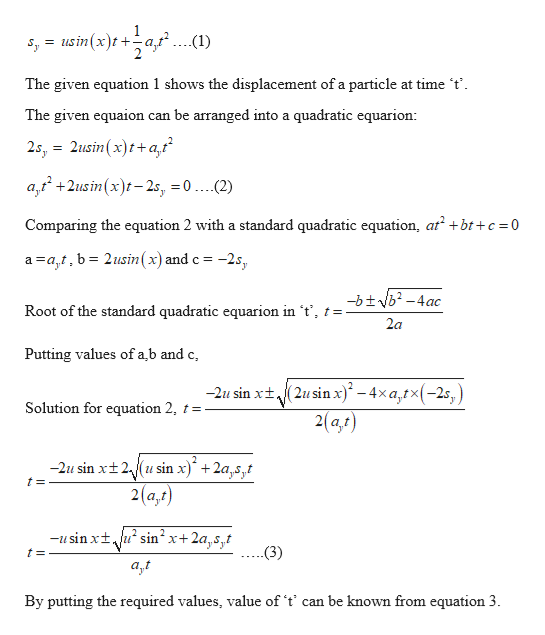How would I solve this equation for t?sy=u sin(x)t+1/2 ayt2

Question

How would I solve this equation for t?

sy=u sin(x)t+1/2 ayt2

Step 1

Given:

sy = u sin(x) t + 1/2 ay t2

Step 2

Showing how to solve the given equation for ‘t’...help_outlineImage Transcriptioncloses usin(x)t- ...(1) = The given equation 1 shows the displacement of a particle at time 't can be arranged into a quadratic equarion The given equaion 2s, 2usin (x)ta,t a2usin (x)t2s, 0 ....(2) + Comparing the equation 2 with a standard quadratic equation, at bt+c 0 a a,t, b= 2usin(x) and c = -2s, -btvb2-4ac Root of the standard quadratic equarion in 't', t = 2a Putting values of a,b and c -2u sin xt(2usinx)*-4xa,tx(-2s,) 2(a,r) Solution for equation 2, t -2u sin xt2usin x) + 2a,s,t 2(a,t) -usin xtusin2 x+2a,s,t .(3) t= By putting the required values, value of 't' can be known from equation 3 fullscreen

Want to see the full answer?

See Solution

Want to see this answer and more?

Our solutions are written by experts, many with advanced degrees, and available 24/7

See Solution
Tagged in

Kinematics